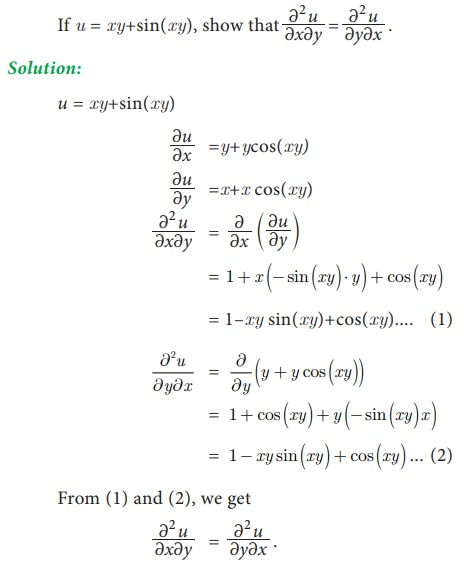Home | | Business Maths 11th std | Eulerâ€™s theorem and its applications

# Eulerâ€™s theorem and its applications

Eulerâ€™s theorem for two variables:

Eulerâ€™s theorem and its applications

Eulerâ€™s theorem for two variables:

If u = f ( x , y)  is a homogeneous function of degree n, thenExample 6.35Example 6.36Example 6.37Example 6.38u is a homogeneous function of degree â€“1

Example 6.39Exercise 6.4Tags : Applications of Differentiation , 11th Business Mathematics and Statistics(EMS) : Chapter 6 : Applications of Differentiation
Study Material, Lecturing Notes, Assignment, Reference, Wiki description explanation, brief detail
11th Business Mathematics and Statistics(EMS) : Chapter 6 : Applications of Differentiation : Eulerâ€™s theorem and its applications | Applications of Differentiation